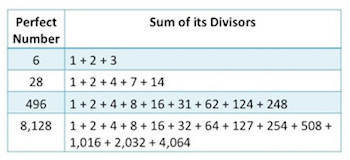# Perfect Numbers: Definition, Formula & Examples

Lesson Transcript
Instructor: Kimberly Osborn
Perfect numbers are positive integers with divisors that, if added together, equal the perfect number. Learn what this means by exploring the definition, formulas, and examples of perfect numbers, including the fifth perfect number. Updated: 10/17/2021

## What Is a Perfect Number?

Since the dawn of mathematics, people have been fascinated with patterns that can be found in the number system. In exploration of these patterns, mathematicians discovered that some numbers actually equal the sum of their divisors (excluding the number itself). Since their first discovery over 2,000 years ago, 48 of these special numbers, called perfect numbers, have been identified. Now, before you roll your eyes at the history lesson I just gave you, I promise it's actually pretty cool once you see some of these perfect numbers in action.

A perfect number is a positive number that equals the sum of its divisors, excluding itself. This is also known as its aliquot sum. At this time, it is unknown how many perfect numbers truly exist in our number system. While we have discovered 48 perfect numbers, the fact that there are an infinite number of prime numbers leads us to believe that there could be an infinite number of perfect numbers. This might seem a little confusing now since we haven't really talked about how prime numbers relate to perfect numbers, but don't worry. It will all make sense once we discuss the formula used to find this pattern and how it applies to finding perfect numbers. It is also interesting to note that all of the perfect numbers that have been discovered are even numbers. Like with the uncertainty in how many perfect numbers exist, it is also uncertain if there are any odd perfect numbers that have just not been found yet.

The first four numbers date back to the time of Euclid over 2,000 years ago. These numbers are 6, 28, 496, and 8,128. This chart shows each perfect number and the sum of its divisors.Looking at the four perfect numbers above, you might begin to notice a pattern. Starting with the divisor of 1, each perfect number begins with a series of powers of 2.

Remember, 1 is the same as 20.

So, looking at our first perfect number, 6, we see 2o, 21, and then 3.

In our second perfect number, 28, we see 20, 21, 22, and then 7.

In our third perfect number, 496, we see 20, 21, 22, 23, 24, and then 31.

This pattern holds true for every perfect number. Each perfect number will begin with a series of powers of 2 followed by a prime number. Now, you might notice something about this prime number. If you look closely, you will see that when you double your last power of 2 and then subtract 1, your result is the prime number!

Take our second perfect number, 28, for example. We see that our last power of 2 is 4, and the prime number that follows is 7. Using the pattern we noted above, doubling our power of 2 (4 x 2) equals 8, minus 1, equals seven.An error occurred trying to load this video.

Try refreshing the page, or contact customer support.

Coming up next: Pyramid in Math: Definition & Practice Problems

### You're on a roll. Keep up the good work!

Replay
Your next lesson will play in 10 seconds
• 0:00 What Is a Perfect Number?
• 1:54 Formula
• 4:57 What Is the Fifth…
• 5:47 Lesson Summary
Save Save

Want to watch this again later?

Timeline
Autoplay
Autoplay
Speed Speed

To unlock this lesson you must be a Study.com Member.

### Register to view this lesson

Are you a student or a teacher?